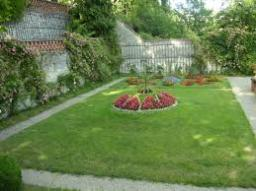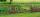# Garden fence

The garden has the shape of a rectangular triangle with an area of 96 square meters and a 16 m long one leg. How many meters of the fence need to be fenced?

x =  48 m

### Step-by-step explanation:

Try calculation via our triangle calculator.Did you find an error or inaccuracy? Feel free to write us. Thank you!Tips to related online calculators
Pythagorean theorem is the base for the right triangle calculator.
Do you want to convert length units?

#### You need to know the following knowledge to solve this word math problem:

We encourage you to watch this tutorial video on this math problem:

## Related math problems and questions:

• Rectangular gardenThe perimeter of Peter's rectangular garden is 98 meters. The width of the garden is 60% shorter than its length. Find the dimensions of the rectangular garden in meters. Find the garden area in square meters.
• GardenThe area of a square garden is 2/9 of triangle garden with sides 160 m, 100 m, and 100 m. How many meters of fencing need to fence a square garden?
• The gardenThe garden has the shape of a rectangular trapezium. The bases have lengths of 27 meters and 36 meters, the trapezoid's height is 12 meters. Calculate how much fence will cost this garden if one meter costs 1.5 €?
• LandRectangular triangular land has area 30 square meters and 12 meters long leg. How many meters of the fence do you need for fencing this land?
• A rectangularA rectangular garden 40 m long and 30 m wide is to be fenced with fence posts at each corner. All the other posts will be 5 meters apart. How many posts will be needed to fence in the garden?
• Triangular landJana has a rectangular garden measuring 30 meters by 72 meters that she wants to split diagonally from corner to corner using a fence. How long does her fence need to be?
• AcreageWhat acreage has a rectangular plot whose diagonal is 34 meters long and one side has a length of 16 meters. ...
• Rectangular gardenThe sides of the rectangular garden are in ratio 1: 2. The diagonal has a length of 20 meters. Calculate the area and perimeter of the garden.
• Horses playgroundThe fence for the horses has the shape of a rectangular trapezoid with an area of 400 m2, the base lengths should be 31 m and 19 m. How many meters of boards will they need to fence it if the boards are stacked in 5 rows?
• GardenRectangular garden 31 meters 40 centimeters long and 20m30cm wide adjacent with the shorter side with another (fenced) garden and on one longest side is 1 meter wide gate. How many meters of fence is needed to buy? (Estimate, calculate, verify a test calc
• Garden GThe rectangular, trapezoidal garden has a base length of 81m, 76m, and a vertical arm of 12m. Calculate how many m2 of the area will remain for planting greenery if 1/3 of the area is built. Calculate the consumption of mesh for land fencing.
• Rectangular triangle PQRIn the rectangular triangle PQR, the PQ leg is divided by the X point into two segments of which longer is 25cm long. The second leg PR has a length 16 cm. The length of the RX is 20 cm. Calculate the length p of side RQ. The result is round to 2 decimal
• Wire fenceThe wire fence around the garden is 160 m long. One side of the garden is three times longer than the other. How many meters do the individual sides of the garden measure?
• GardensThe area of the square garden is 3/4 of the area of the triangular garden with sides of 80 m, 50 m, 50 m. How many meters of the fence do we need to fence a square garden?
• Free space in the gardenThe grandfather's free space in the garden was in the shape of a rectangular triangle with 5 meters and 12 meters in length. He decided to divide it into two parts and the height of the hypotenuse. For the smaller part creates a rock garden, for the large
• R TrapeziumRectangular trapezium has bases 21 and 10 and area 92 cm2. What is its perimeter?
• Rectangular gardenRectangular garden has a length of 48.7 m, a width of 6.3 meters shorter than the length. How much mesh should be bought for its fencing if the gate is 2.9 m long and the gate 1.1 m? What is the area of the garden?